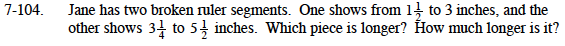### Home > MC1 > Chapter 7 > Lesson 7.3.1 > Problem7-104

7-104.You can use subtraction to find the length of each segment and then you can compare them.

Find the length of the first segment.

$3-1\frac{1}{2}=3\frac{2}{2}\minus\frac{3}{2}=\frac{6}{2}-\frac{3}{2}=\frac{3}{2}=1\frac{1}{2}\text{ inches}$

Find the length of the second segment.

$5\frac{1}{2}\minus3\frac{1}{4}=\frac{11}{2}\minus\frac{13}{4}=\frac{22}{4}\minus\frac{13}{4}=\frac{9}{4}=2\frac{1}{4} \text{ inches}$

The second segment is longer. Use subtraction to figure out the difference in their lengths.